# Reversible Pendulum - Kater's pendulum

\$250.00

Code Number: Updating

Brand: AELAB

#### Purpose:

Determining the gravitational acceleration with a reversible pendulum

- +

## Including:

- Reversible Pendulum: Length L = 120cm, Distance between 2 edges d = 80cm. There are 25 lines in Stainless steel rod, 2.5cm apart. There are 2 weights with m1 = 940g and m2 = 1360g. Edge holder is wall attachable.

- Ruller 30cm

#### Objects of the experiments.

- Measuring the oscillation periods T1 and T2 of a reversible pendulum for two suspension points.

- Tuning the reversible pendulum to the same oscillation period.

- Determining the gravitational acceleration from the oscillation period and the reduced length of pendulum.

## Theory:

With compound pendulum, the oscillation formula: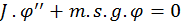(1)

J:  Moment of inertia of pendulum via oscillation axis (H1 or H2).

s: Distance from weight (m2) to oscillation axis (H1 or H2).

g:  Gravitational acceleration.

m: Mass of pendulum.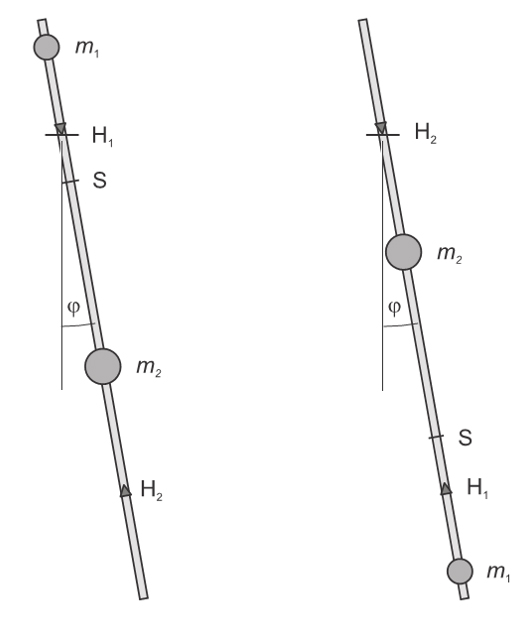The reduced length Sr of the compound pendulum is defined as the quantity: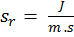(2)

because its oscillation period: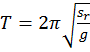(3)

corresponds to that of a simple pendulum with the length Sr.
The moment of inertia J of the compound pendulum is, according to the parallel axis theorem: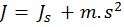(4)

Js: moment of inertia around the centre of mass axis.

Therefore the reduced length of pendulum is: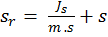(5)

#### With reversible pendulum:

The reversible pendulum is a particular type of the compound pendulum. There are two edges H1 and H2 that allow to choose the suspension point.. Two weights m1 = 940g và m2 = 1360g can be shifted so that the oscillation period is tunable. The goal of the tuning is to achieve equal oscillation periods around both edges

When T1 = T2, In this case, the reduced length of pendulum is equal: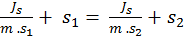(6)

s1 and  s2 is distance from weight m2 to H1 anf H2. Then: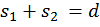(7)

d: distance from H1 to H2.

From formula (6) and (7):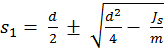(8)

S1 is inserted in Eq (5):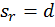(9)

Then: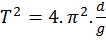(10)

Set up and procedure: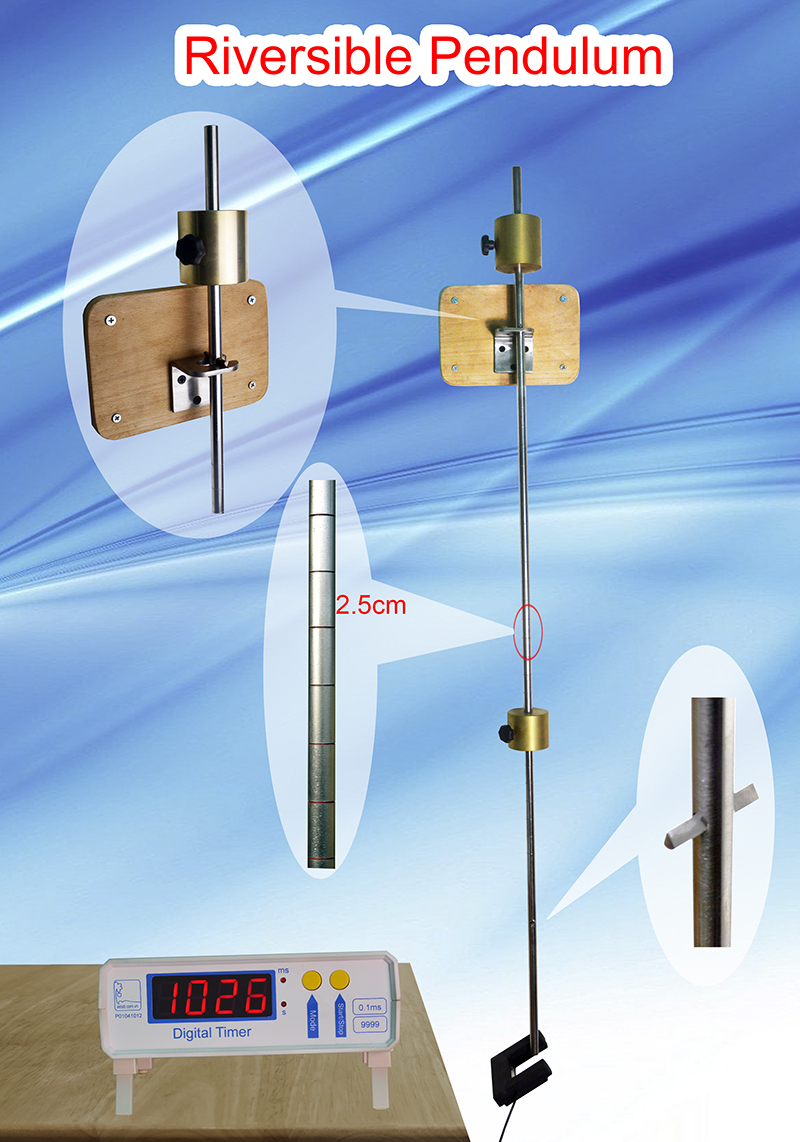Reversible Pendulum (Kater's pendulum)

- Mount the wall holder on a stable, non-vibrating wall.

- Attach photogate to wall (by screw), so that pendulum scan to IR eye of photogate (see the clip below)

- Fix the mass m1 at the position x1 = 10 cm from H1 (position of m1 can be changed)

- We do experiment with m2 at position x2 = 9.7; 12.2; 14.7 cm; .... 69.7cm from H1 (the top line of pendulum is away from axis of oscillation H1 9.7cm)

Measure period by 2 ways:

+ The first way: Select Mode 4 in photogate timer. To measure period in directly.

+ Second way: Select Mode 5 in photogate timer: Count 50 periods, measure total time. Then calculate average period.

We recommend the second way for better accuracy.

- Deflect the pendulum a small angle, the rod of reversible pendulum cover IR eye of photogate. Release the rod, the Kater's pendulum will oscillate.

- Press Start/Stop button to count. Wait for counting 50 periods then press Start/Stop again to stop count.

- Write the total time for 50 periods to data table.

- Slide the weight m2 to second line position (x2 = 12.2cm). Measure 50 periods again.

- After we do with the 25th line (x2 = 69.7cm). We reverse the pendulum, the axis of oscillation is H2.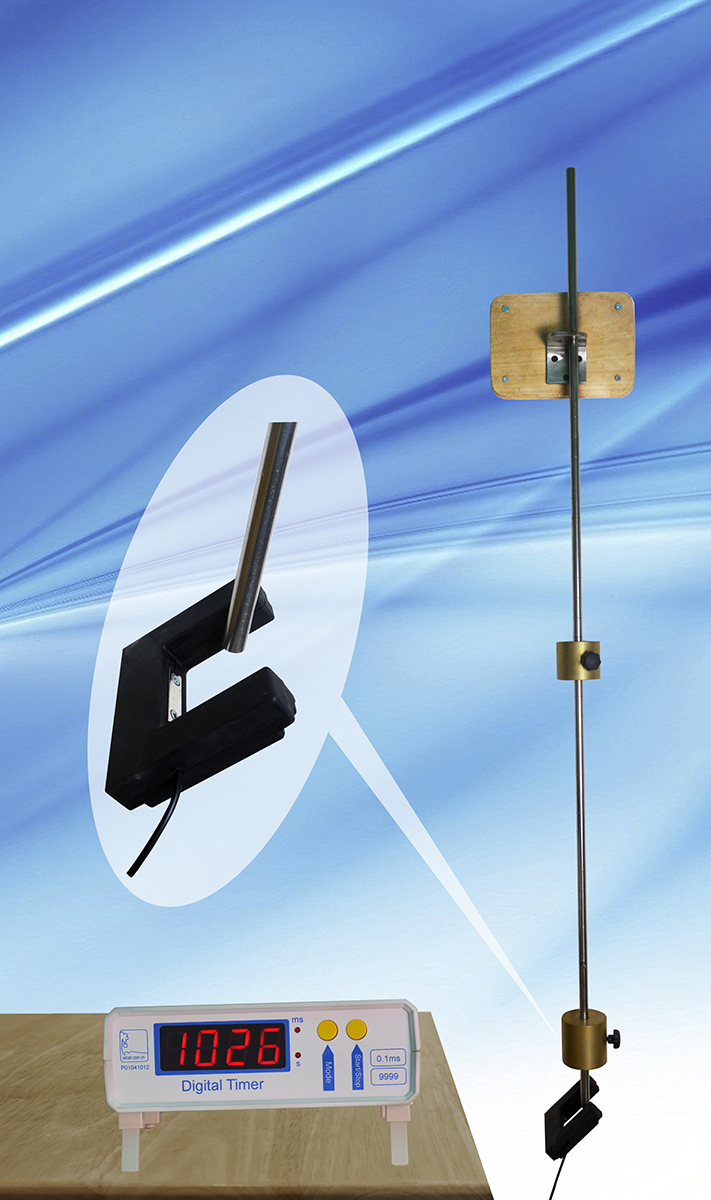Reversible Pendulum: Axis H2

- We do experiment again similar to doing with axis H1.

- We have the experiment data below: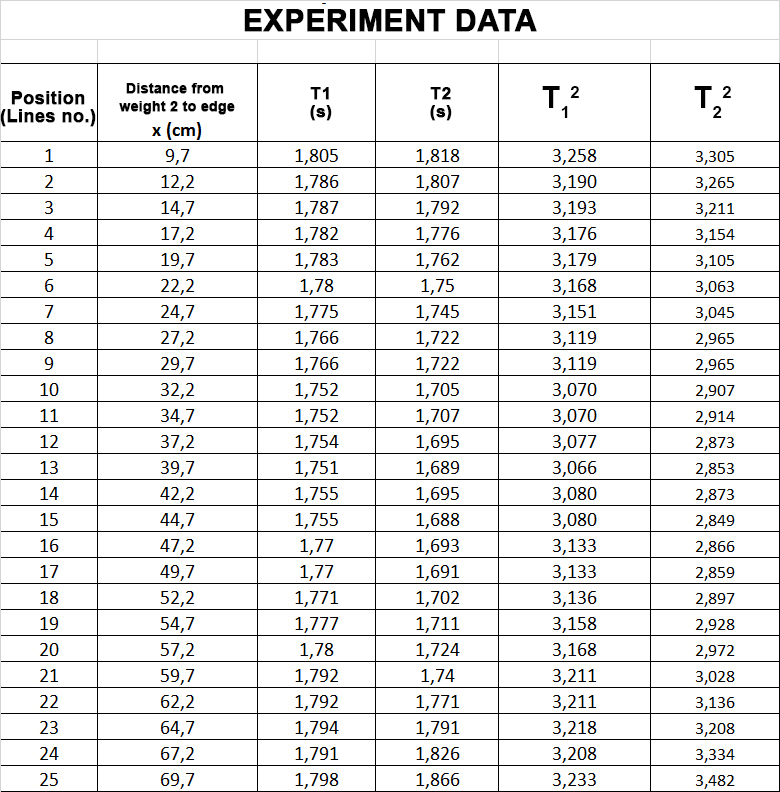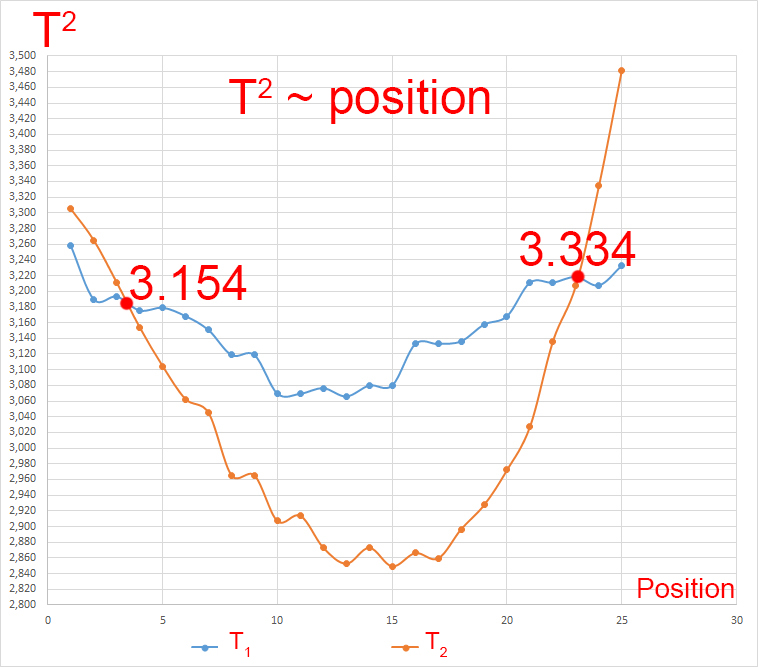Use this formula to determine the gravitational acceleration:(10)

The graphs T1 and T2 intersect  at: 3.154 and 3.334

From formula (10) above, so g1 = 10m/s2, g2 =  9.46m/s2

Average value: g = 9.73m/s2

Error: 0.82%